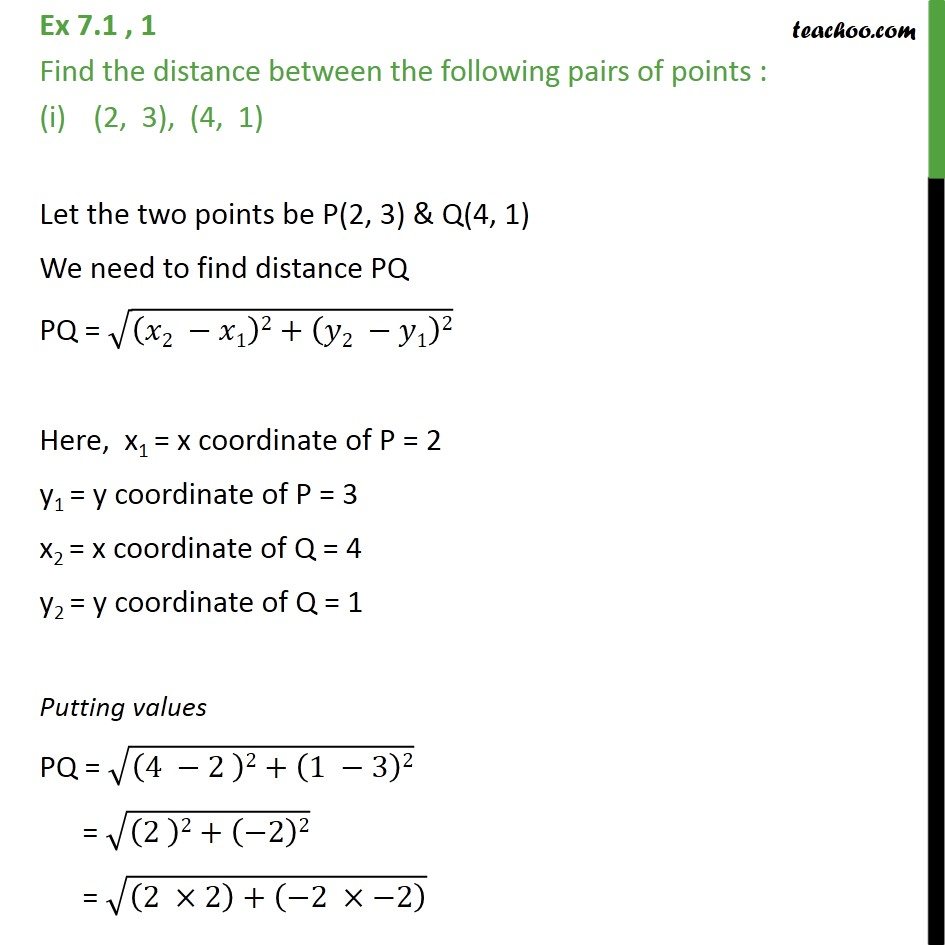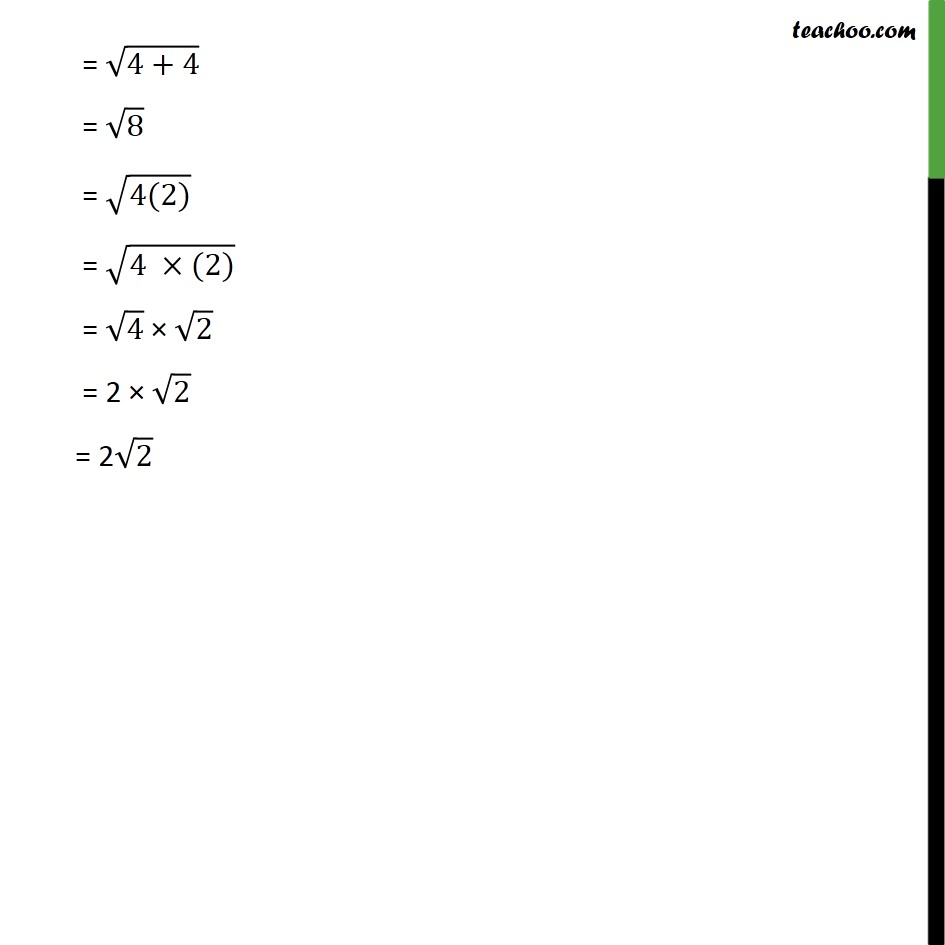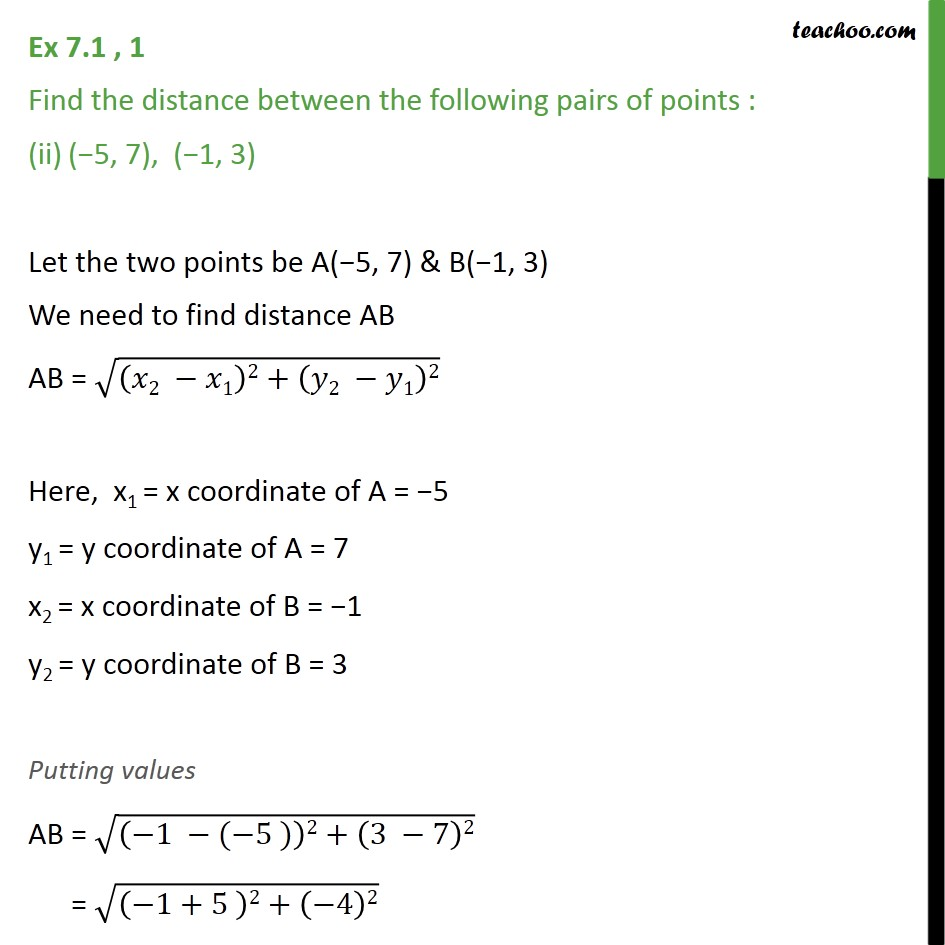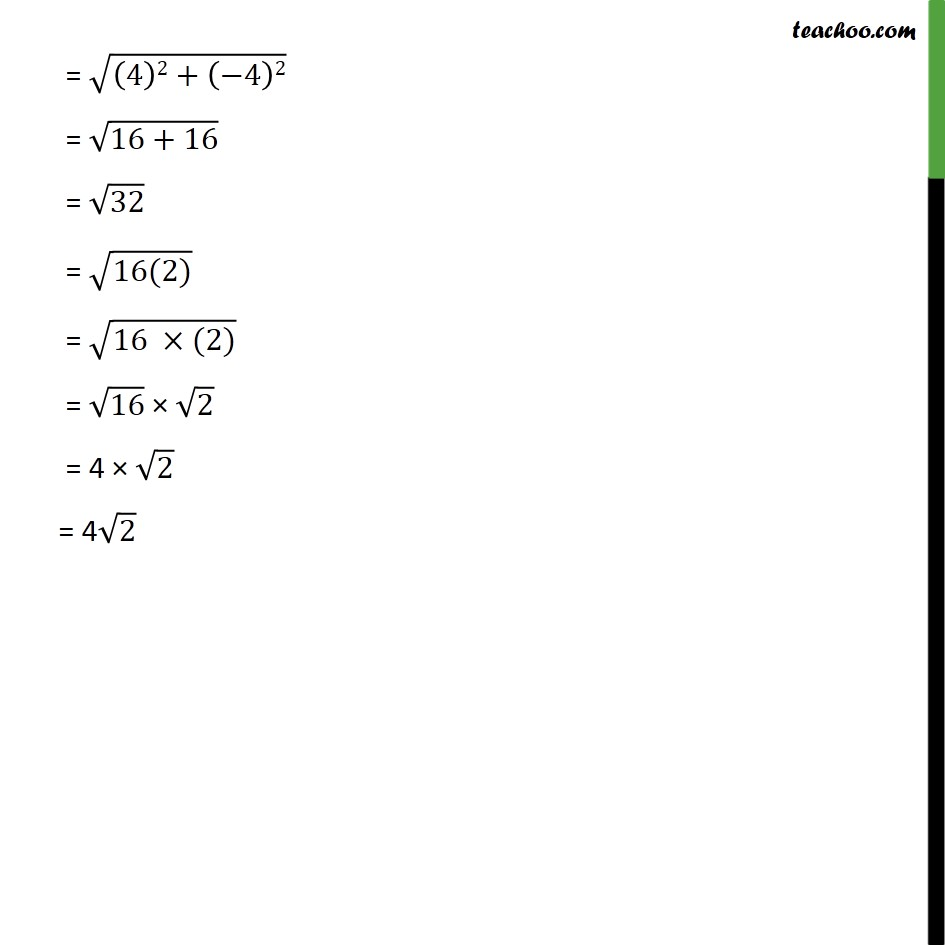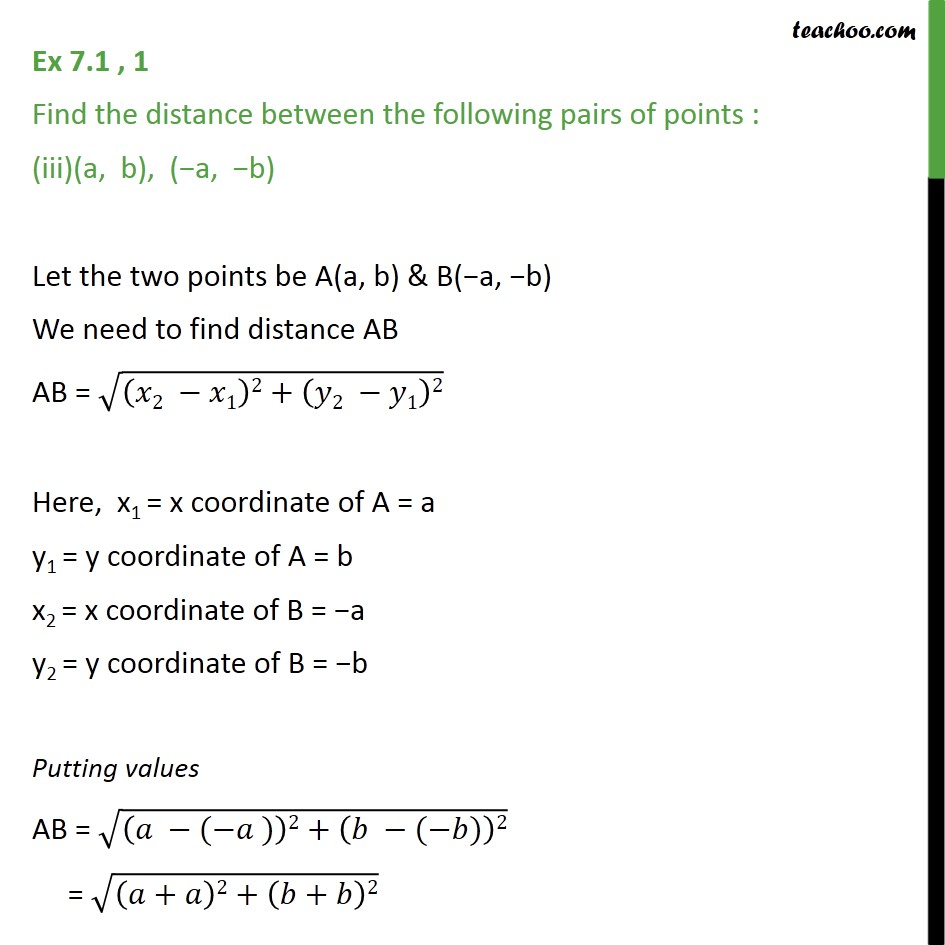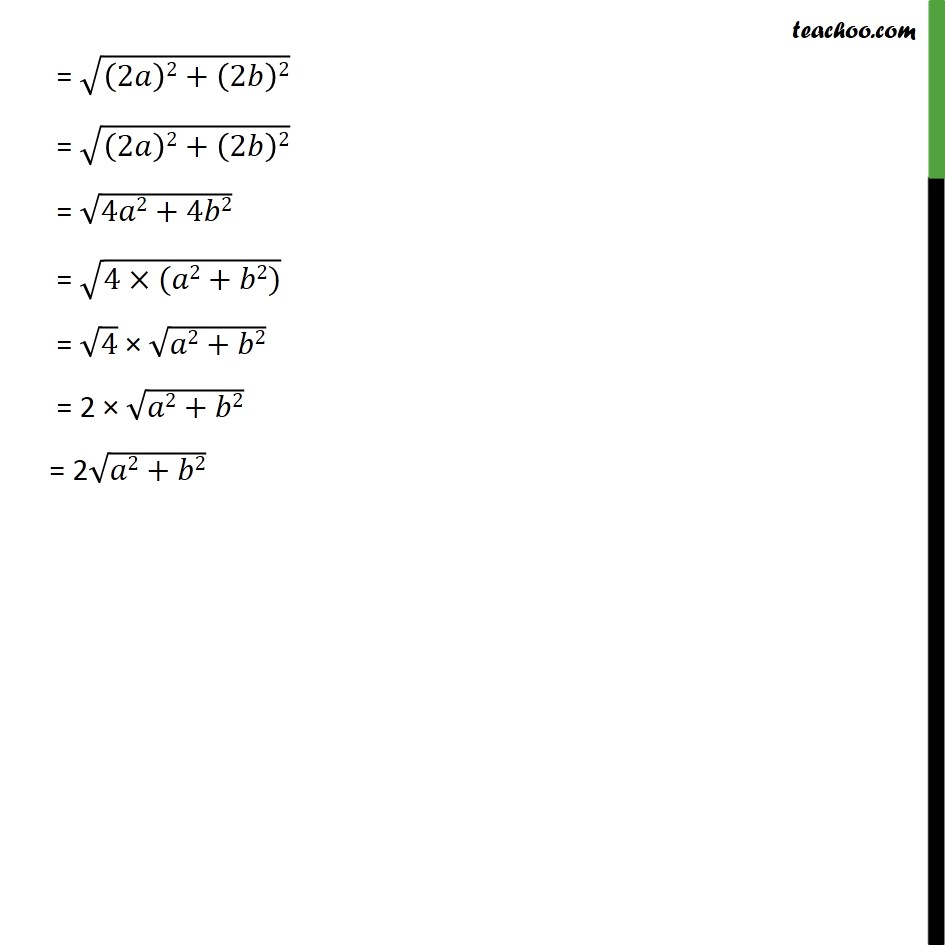1. Chapter 7 Class 10 Coordinate Geometry
2. Serial order wise
3. Ex 7.1

Transcript

Ex 7.1 , 1 Find the distance between the following pairs of points : (2, 3), (4, 1) Let the two points be P(2, 3) & Q(4, 1) We need to find distance PQ PQ = (( 2 1)2+( 2 1)2) Here, x1 = x coordinate of P = 2 y1 = y coordinate of P = 3 x2 = x coordinate of Q = 4 y2 = y coordinate of Q = 1 Putting values PQ = ((4 2 )2+(1 3)2) = ((2 )2+( 2)2) = ((2 2)+( 2 2) ) = (4+4) = 8 = (4(2)) = (4 (2)) = 4 2 = 2 2 = 2 2 Ex 7.1 , 1 Find the distance between the following pairs of points : ( 5, 7), ( 1, 3) Let the two points be A( 5, 7) & B( 1, 3) We need to find distance AB AB = (( 2 1)2+( 2 1)2) Here, x1 = x coordinate of A = 5 y1 = y coordinate of A = 7 x2 = x coordinate of B = 1 y2 = y coordinate of B = 3 Putting values AB = (( 1 ( 5 ))2+(3 7)2) = (( 1+5 )2+( 4)2) = ((4)2+( 4)2) = (16+16) = 32 = (16(2)) = (16 (2)) = 16 2 = 4 2 = 4 2 Ex 7.1 , 1 Find the distance between the following pairs of points : (a, b), ( a, b) Let the two points be A(a, b) & B( a, b) We need to find distance AB AB = (( 2 1)2+( 2 1)2) Here, x1 = x coordinate of A = a y1 = y coordinate of A = b x2 = x coordinate of B = a y2 = y coordinate of B = b Putting values AB = (( ( ))2+( ( ))2) = (( + )2+( + )2) = ((2 )2+(2 )2) = ((2 )2+(2 )2) = (4 2+4 2) = (4 ( 2+ 2)) = 4 ( 2+ 2) = 2 ( 2+ 2) = 2 ( 2+ 2)

Ex 7.1